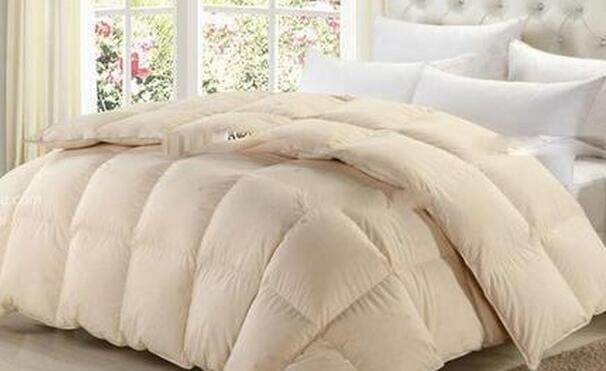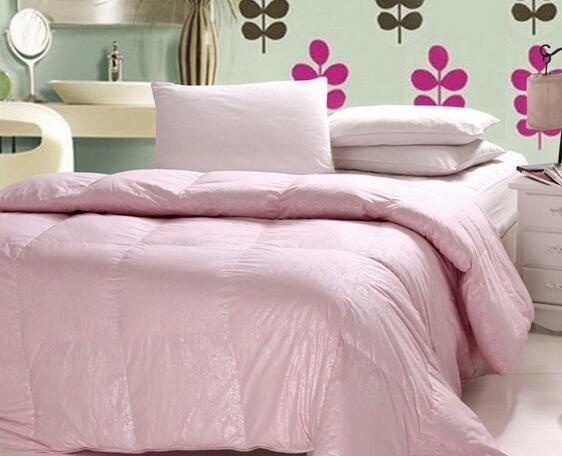|

# 百思寒羽绒床品品质怎么样 质量如何?

百思寒羽绒床品在这个冬季一直以来都是市场上畅销冠军，作为性价比之王，业内知名床上用品品牌，百思寒羽绒床品花色和款式众多与其它床品相比独树一帜，备受人们青睐，那百思寒羽绒床品品质怎么样，质量如何？百思寒羽绒床品之产品

百思寒羽绒床品花色和款式众多，面料上等，填充以高冬天蓬松的优质羽绒，轻柔舒适，保暖效果极好，让人不必在为寒冷担忧，度过一个温暖的冬天。而且百思寒采用的是网上销售，节省了很多费用，使得百思寒羽绒床品价格非常实惠，同等品质产品的价格只有商场的三分之一。

百思寒羽绒床品之质量

百思寒羽绒床品采用先进的原料处理和防跑绒技术，环保无异味，质量很好，已全面通过ISO900国际质量体系认证，而且全部产品都有防伪商标，顾客可免费拨打800或400进行查证，让顾客买得更放心。

百思寒羽绒床品之服务

百思寒羽绒床品是淘宝认证的信誉商家单位，有正品保证，假一罚三，并支持七天无理由退换货，一切以客户的满意度为先，服务热情周到，品质和服务得到数万名顾客的见证，回头购买率达九成以上。

百思寒羽绒床品款式众多，品质一流，服务周到，性价比高，让你远离寒冷的侵袭，是购买的良好选择。百思寒羽绒床品之

网友评价

jusuan：这是我买的第三床被了，真是没的说，太好了，很温暖，质量好，做工也好，聚划算买的，狠狠是，优惠不少，好品牌值得信赖，包装也好，细致物有所值。包装得很紧扎，拿出来后在太阳下晒晒，拍打拍打就鼓起来了。爷爷很满意说这被子既轻巧又保暖。

流年还是榴莲：我是买了13天，延长收货时间以后来评价的，客服态度很好，质量也很好，没有什么异味，几天过后被子会膨松厚实起来，给婆婆和一岁的宝宝用的，很满意。

suan：第一次买羽绒被，不错，很暖和，轻轻的，比超市的便宜，值得拥有。没有味道。

幼稚园的小朋友：去年买过此品牌的羽绒服，质量特别好。后来开始关注此家的羽绒被，这次一下子买了3床，晒后蓬松没味道。超级赞！

床上用品注意保养

1、床上用品（不含芯类），清洗频率可根据个人的卫生习惯而定。初次使用前，可先下水漂洗一次，可将表面的浆质及印染浮色洗掉，使用起来会比较柔软，将来清洗时也不大容易褪色。

2、除了较特殊的材质以及注明不能水洗者（如真丝），一般而言，洗涤程序为：先将中性洗涤剂倒入洗衣机的水中，水温不要超过30℃，待洗涤剂完全溶解后再放入床上用品，浸泡的时间不要太久。因为使用碱性洗涤剂或水温太高或洗涤剂没有平均溶解或浸泡太久都可能造成不必要的褪色情形。同时，清洗时浅色产品要与深色产品分开洗涤，避免互相染色。清洗后在室外通风处晾干即可，若要使用烘干机，请选用低温烘干，温度不要超过35℃，可以避免它过度缩水。床上用品一般是摆放于床上，供人在睡眠时使用的物品，包括被褥、被套、床单、床罩、床笠、枕套、枕芯、毯子、凉席和蚊帐；一般，我们所指的床上用品主要指纺织制品、绗缝制品和聚酯纤维制品，不包括毯子和凉席。

总之，清洗前应仔细阅读有关产品的洗涤说明，有装饰附件的产品洗涤前一定要注意把花边、坠子等先取下，避免损坏。

3、收藏时请先清洗干净，彻底晾干，折叠整齐，并放入一定量的樟脑丸（不能与产品直接接触），宜放在暗处、湿度低、通风良好的地方。长期不使用的被类产品在重新使用前可先在阳光下晾晒，使其恢复蓬松。

4、特别注意事项：

A、亚麻产品洗涤时不能用力搓、拧（因为纤维较脆，易起毛，影响外观和寿命）。

B、棉、麻产品收藏时要注意保持环境清洁干净，防止霉变。浅色和深色的产品要注意分开存放，防止影色、泛黄。

C、白色真丝产品不能放樟脑丸或放在樟木箱内，否则会泛黄。

D、除单孔纤维枕外，其他均可洗涤，但因其有厚度，一定要保证充分晾晒，使其完全干燥才不影响再次使用。平时最好使用枕套，免去洗涤的麻烦。

`声明：本文由入驻焦点开放平台的作者撰写，除焦点官方账号外，观点仅代表作者本人，不代表焦点立场错误信息举报电话： 400-099-0099，邮箱：jubao@vip.sohu.com，或点此进行意见反馈，或点此进行举报投诉。`A B C D E F G H J K L M N P Q R S T W X Y Z
A - B - C - D - E
• A
• 鞍山
• 安庆
• 安阳
• 安顺
• 安康
• 澳门
• B
• 北京
• 保定
• 包头
• 巴彦淖尔
• 本溪
• 蚌埠
• 亳州
• 滨州
• 北海
• 百色
• 巴中
• 毕节
• 保山
• 宝鸡
• 白银
• 巴州
• C
• 承德
• 沧州
• 长治
• 赤峰
• 朝阳
• 长春
• 常州
• 滁州
• 池州
• 长沙
• 常德
• 郴州
• 潮州
• 崇左
• 重庆
• 成都
• 楚雄
• 昌都
• 慈溪
• 常熟
• D
• 大同
• 大连
• 丹东
• 大庆
• 东营
• 德州
• 东莞
• 德阳
• 达州
• 大理
• 德宏
• 定西
• 儋州
• 东平
• E
• 鄂尔多斯
• 鄂州
• 恩施
F - G - H - I - J
• F
• 抚顺
• 阜新
• 阜阳
• 福州
• 抚州
• 佛山
• 防城港
• G
• 赣州
• 广州
• 桂林
• 贵港
• 广元
• 广安
• 贵阳
• 固原
• H
• 邯郸
• 衡水
• 呼和浩特
• 呼伦贝尔
• 葫芦岛
• 哈尔滨
• 黑河
• 淮安
• 杭州
• 湖州
• 合肥
• 淮南
• 淮北
• 黄山
• 菏泽
• 鹤壁
• 黄石
• 黄冈
• 衡阳
• 怀化
• 惠州
• 河源
• 贺州
• 河池
• 海口
• 红河
• 汉中
• 海东
• I
• J
• 晋中
• 锦州
• 吉林
• 鸡西
• 佳木斯
• 嘉兴
• 金华
• 景德镇
• 九江
• 吉安
• 济南
• 济宁
• 焦作
• 荆门
• 荆州
• 江门
• 揭阳
• 金昌
• 酒泉
• 嘉峪关
K - L - M - N - P
• K
• 开封
• 昆明
• 昆山
• L
• 廊坊
• 临汾
• 辽阳
• 连云港
• 丽水
• 六安
• 龙岩
• 莱芜
• 临沂
• 聊城
• 洛阳
• 漯河
• 娄底
• 柳州
• 来宾
• 泸州
• 乐山
• 六盘水
• 丽江
• 临沧
• 拉萨
• 林芝
• 兰州
• 陇南
• M
• 牡丹江
• 马鞍山
• 茂名
• 梅州
• 绵阳
• 眉山
• N
• 南京
• 南通
• 宁波
• 南平
• 宁德
• 南昌
• 南阳
• 南宁
• 内江
• 南充
• P
• 盘锦
• 莆田
• 平顶山
• 濮阳
• 攀枝花
• 普洱
• 平凉
Q - R - S - T - W
• Q
• 秦皇岛
• 齐齐哈尔
• 衢州
• 泉州
• 青岛
• 清远
• 钦州
• 黔南
• 曲靖
• 庆阳
• R
• 日照
• 日喀则
• S
• 石家庄
• 沈阳
• 双鸭山
• 绥化
• 上海
• 苏州
• 宿迁
• 绍兴
• 宿州
• 三明
• 上饶
• 三门峡
• 商丘
• 十堰
• 随州
• 邵阳
• 韶关
• 深圳
• 汕头
• 汕尾
• 三亚
• 三沙
• 遂宁
• 山南
• 商洛
• 石嘴山
• T
• 天津
• 唐山
• 太原
• 通辽
• 铁岭
• 泰州
• 台州
• 铜陵
• 泰安
• 铜仁
• 铜川
• 天水
• 天门
• W
• 乌海
• 乌兰察布
• 无锡
• 温州
• 芜湖
• 潍坊
• 威海
• 武汉
• 梧州
• 渭南
• 武威
• 吴忠
• 乌鲁木齐
X - Y - Z
• X
• 邢台
• 徐州
• 宣城
• 厦门
• 新乡
• 许昌
• 信阳
• 襄阳
• 孝感
• 咸宁
• 湘潭
• 湘西
• 西双版纳
• 西安
• 咸阳
• 西宁
• 仙桃
• 西昌
• Y
• 运城
• 营口
• 盐城
• 扬州
• 鹰潭
• 宜春
• 烟台
• 宜昌
• 岳阳
• 益阳
• 永州
• 阳江
• 云浮
• 玉林
• 宜宾
• 雅安
• 玉溪
• 延安
• 榆林
• 银川
• Z
• 张家口
• 镇江
• 舟山
• 漳州
• 淄博
• 枣庄
• 郑州
• 周口
• 驻马店
• 株洲
• 张家界
• 珠海
• 湛江
• 肇庆
• 中山
• 自贡
• 资阳
• 遵义
• 昭通
• 张掖
• 中卫

1室1厅1厨1卫1阳台

1
2
3
4
5

0
1
2

1

1

0
1
2
3报名成功，资料已提交审核A B C D E F G H J K L M N P Q R S T W X Y Z
A - B - C - D - E
• A
• 鞍山
• 安庆
• 安阳
• 安顺
• 安康
• 澳门
• B
• 北京
• 保定
• 包头
• 巴彦淖尔
• 本溪
• 蚌埠
• 亳州
• 滨州
• 北海
• 百色
• 巴中
• 毕节
• 保山
• 宝鸡
• 白银
• 巴州
• C
• 承德
• 沧州
• 长治
• 赤峰
• 朝阳
• 长春
• 常州
• 滁州
• 池州
• 长沙
• 常德
• 郴州
• 潮州
• 崇左
• 重庆
• 成都
• 楚雄
• 昌都
• 慈溪
• 常熟
• D
• 大同
• 大连
• 丹东
• 大庆
• 东营
• 德州
• 东莞
• 德阳
• 达州
• 大理
• 德宏
• 定西
• 儋州
• 东平
• E
• 鄂尔多斯
• 鄂州
• 恩施
F - G - H - I - J
• F
• 抚顺
• 阜新
• 阜阳
• 福州
• 抚州
• 佛山
• 防城港
• G
• 赣州
• 广州
• 桂林
• 贵港
• 广元
• 广安
• 贵阳
• 固原
• H
• 邯郸
• 衡水
• 呼和浩特
• 呼伦贝尔
• 葫芦岛
• 哈尔滨
• 黑河
• 淮安
• 杭州
• 湖州
• 合肥
• 淮南
• 淮北
• 黄山
• 菏泽
• 鹤壁
• 黄石
• 黄冈
• 衡阳
• 怀化
• 惠州
• 河源
• 贺州
• 河池
• 海口
• 红河
• 汉中
• 海东
• I
• J
• 晋中
• 锦州
• 吉林
• 鸡西
• 佳木斯
• 嘉兴
• 金华
• 景德镇
• 九江
• 吉安
• 济南
• 济宁
• 焦作
• 荆门
• 荆州
• 江门
• 揭阳
• 金昌
• 酒泉
• 嘉峪关
K - L - M - N - P
• K
• 开封
• 昆明
• 昆山
• L
• 廊坊
• 临汾
• 辽阳
• 连云港
• 丽水
• 六安
• 龙岩
• 莱芜
• 临沂
• 聊城
• 洛阳
• 漯河
• 娄底
• 柳州
• 来宾
• 泸州
• 乐山
• 六盘水
• 丽江
• 临沧
• 拉萨
• 林芝
• 兰州
• 陇南
• M
• 牡丹江
• 马鞍山
• 茂名
• 梅州
• 绵阳
• 眉山
• N
• 南京
• 南通
• 宁波
• 南平
• 宁德
• 南昌
• 南阳
• 南宁
• 内江
• 南充
• P
• 盘锦
• 莆田
• 平顶山
• 濮阳
• 攀枝花
• 普洱
• 平凉
Q - R - S - T - W
• Q
• 秦皇岛
• 齐齐哈尔
• 衢州
• 泉州
• 青岛
• 清远
• 钦州
• 黔南
• 曲靖
• 庆阳
• R
• 日照
• 日喀则
• S
• 石家庄
• 沈阳
• 双鸭山
• 绥化
• 上海
• 苏州
• 宿迁
• 绍兴
• 宿州
• 三明
• 上饶
• 三门峡
• 商丘
• 十堰
• 随州
• 邵阳
• 韶关
• 深圳
• 汕头
• 汕尾
• 三亚
• 三沙
• 遂宁
• 山南
• 商洛
• 石嘴山
• T
• 天津
• 唐山
• 太原
• 通辽
• 铁岭
• 泰州
• 台州
• 铜陵
• 泰安
• 铜仁
• 铜川
• 天水
• 天门
• W
• 乌海
• 乌兰察布
• 无锡
• 温州
• 芜湖
• 潍坊
• 威海
• 武汉
• 梧州
• 渭南
• 武威
• 吴忠
• 乌鲁木齐
X - Y - Z
• X
• 邢台
• 徐州
• 宣城
• 厦门
• 新乡
• 许昌
• 信阳
• 襄阳
• 孝感
• 咸宁
• 湘潭
• 湘西
• 西双版纳
• 西安
• 咸阳
• 西宁
• 仙桃
• 西昌
• Y
• 运城
• 营口
• 盐城
• 扬州
• 鹰潭
• 宜春
• 烟台
• 宜昌
• 岳阳
• 益阳
• 永州
• 阳江
• 云浮
• 玉林
• 宜宾
• 雅安
• 玉溪
• 延安
• 榆林
• 银川
• Z
• 张家口
• 镇江
• 舟山
• 漳州
• 淄博
• 枣庄
• 郑州
• 周口
• 驻马店
• 株洲
• 张家界
• 珠海
• 湛江
• 肇庆
• 中山
• 自贡
• 资阳
• 遵义
• 昭通
• 张掖
• 中卫• 手机• 分享
• 设计
免费设计
• 计算器
装修计算器
• 入驻
合作入驻
• 联系
联系我们
• 置顶
返回顶部